## Table of ContentOpen Access

ARTICLE

# Aggregation Operators for Decision Making Based on q-Rung Orthopair Fuzzy Hypersoft Sets: An Application in Real Estate Project

Salma Khan1, Muhammad Gulistan1, Nasreen Kausar2, Dragan Pamucar3, Tzung-Pei Hong4,5, Hafiz Abdul Wahab1,*

1 Department of Mathematics and Statistics, Hazara University Mansehra, 21120, Pakistan
2 Department of Mathematics, Faculty of Arts and Sciences, Yildiz Technical University, Esenler, Istanbul, 34210, Turkey
4 Department of Computer Science and Information Engineering, National University of Kaohsiung, Kaohsiung, 804, Taiwan
5 Department of Computer Science and Engineering, National Sun Yat-sen University, Kaohsiung, 804, Taiwan

* Corresponding Author: Hafiz Abdul Wahab. Email:(This article belongs to this Special Issue: Advanced Computational Models for Decision-Making of Complex Systems in Engineering)

Computer Modeling in Engineering & Sciences 2023, 136(3), 3141-3156. https://doi.org/10.32604/cmes.2023.026169

## Abstract

In this paper, a decision-making problem with a q-rung orthopair fuzzy hypersoft environment is developed, and two operators of ordered weighted average and induced ordered weighted average are developed. Several fundamental features are also derived. The induced ordered weighted average operator is essential in a q-ROFH environment as the induced ordered aggregation operators are special cases of the existing aggregation operators that already exist in q-ROFH environments. The main function of these operators is to help decision-makers gain a complete understanding of uncertain facts. The proposed aggregation operator is applied to a decision-making problem, with the aim of selecting the most promising real estate project for investment.

## Graphical Abstract## Keywords

1  Introduction

Decision making  is a crucial aspect of our daily lives. Due to the complexity and subjectivity of complex alternatives, decision-makers are frequently confronted with a considerable measure of ambiguity in decision-making problems. To address these problems, Zadeh  developed fuzzy set theory. Each element of such set is assigned a membership value, between 0 and 1. The fuzzy set theory has a significant impact on the decision-making process. The fuzzy set theory has numerous applications in numerous domains of engineering, humanities, health, and physical sciences. In recent years, fuzzy set theory has also been utilized in the fields of energy, medicine, materials, economics, and nearly every other scientific discipline. There must be uncertainty if the decision-makers do not have sufficient knowledge to deal with the data necessary to express their preference for the object. Experts mostly consider membership and non-membership values in the decision-making process that the fuzzy set cannot handle. Using the framework of fuzzy multisets, Du et al.  developed a multicriteria group decision-making method in 2021, which accounts for varying degrees of risk associated with each criterion.

To deal with this type of circumstance, Atanassov [4,5] proposed intuitionistic fuzzy sets, whose primary components are intuitionistic fuzzy numbers (IFN). Intuitionistic fuzzy numbers are defined by a membership function and a non-membership function, with the condition that the sum of the membership and non-membership grades is less than or equal to one. When the sum is greater than 1, the intuitionistic fuzzy sets do not work under specific conditions. Several operations on intuitionistic fuzzy sets were developed by De et al. [6,7] and incorporated into Sanchez’s method for medical diagnosis. The distance between intuitionistic fuzzy sets was discussed by Szmidt et al. . Using intuitionistic fuzzy sets as a theme, Gulzar et al.  introduced the t-intuitionistic fuzzy subgroup with basic algebraic properties and complex intuitionistic fuzzy sets in group theory and its applications. In some instances, when the sum of the degrees of MM and NMM is greater than 1, their sum of squares may be less than or equal to 1. Intuitionistic fuzzy sets fail in this situation.

To address these problems, Yager  proposed a novel concept known as the Pythagorean fuzzy set, a generalization of the intuitionistic fuzzy sets. The quadratic sum of membership and non-membership is less than or equal to 1, distinguishing it from other entities. Intuitionistic fuzzy sets and Pythagorean fuzzy sets are incredibly valuable for characterising fuzzy data. It is obvious that the Pythagorean fuzzy set can represent more decision-making information and is more inclusive than the intuitionistic fuzzy set. However, the Pythagorean fuzzy set frequently fails when the decision-maker is able to specify the degree of the membership and non-membership in a particular case where their sum is greater than 1. Some findings for Pythagorean fuzzy sets were developed in 2015 . Pythagorean fuzzy sets, as well as a more refined approach to linguistic, Garg  utilized Pythagorean fuzzy sets for MCDM problems.

Yager  came up with q-rung orthopair fuzzy sets (q-ROFS), the key feature of which is that the sum of the qth power of the membership and non-membership grade cannot exceed 1. Because of this, q-ROFS is advantageous to both the intuitionistic fuzzy set and the Pythagorean fuzzy set when dealing with ambiguous data. Innovative applications of fuzzy sets include aggregation operators, information measures, and decision-making tools. Madasi et al.  recently proposed the topic of N-cubic q-rung orthopair fuzzy sets, along with their properties and the use of mobile apps in the education sector as an application.

In order to address the issue of making group decisions based on several criteria, Zulqarnain et al.  developed certain aggregation operators inside the framework of the Interval-Valued Pythagorean fuzzy soft set. After transforming the function F into a multi-valued function, Smarandache  introduced the hypersoft set. Hypersoft sets are a fundamental mathematical tool for dealing with ambiguity and uncertainty in problem-based decision-making. Zulqarnain et al. [17,18] developed the Pythagorean fuzzy hypersoft sets, along with fundamental operations and techniques. By combining these concepts, Khan et al.  constructed a new structure called q-rung orthopair fuzzy hypersoft sets (q-ROFHSs). In q-ROFHSs, the multi-parameter membership and non-membership grades are limited to the qth power.

It is crucial to understand the aggregation operators in the q-rung orthopair fuzzy environment and other types of fuzzy sets for tackling problems involving decision-making. In order to solve the decision-making techniques, the induced intuitionistic fuzzy ordered weighted geometric (I-IFOWG) and induced interval-valued intuitionistic fuzzy ordered weighted geometric (I-IIFOWG) operators were developed by Wei . The induced generalised intuitionistic fuzzy ordered weighted averaging (I-GIFOWA) and induced generalised interval-valued intuitionistic fuzzy ordered weighted averaging (I-GIIFOWA) operators were introduced by Xu et al. . For the sake of better decision-making, Zulqarnain et al.  also explored a variety of aggregation operators in a Pythagorean fuzzy hypersoft environment. Basic aggregation operators, such as the weighted average and weighted geometric operators were designed by Liu et al. , and they were applied in a scenario based on q-rung orthopair fuzzy information. Several fundamental operations and exponential aggregation operators were developed by Peng et al.  for the q-rung orthopair fuzzy set. Two aggregation operators the complex q-rung orthopair fuzzy weighted average (Cq-ROFWA) and the complex q-rung orthopair fuzzy weighted geometric (Cq-ROFWG) were introduced by Liu et al. . For a complex q-rung orthopair fuzzy (Cq-ROF) environment, Garg et al.  developed power aggregation operations. The q-rung orthopair fuzzy hypersoft weighted average (q-ROFHWA) and q-rung orthopair fuzzy hypersoft weighted geometric (q-ROFHWG) aggregation operators were developed by Khan et al.  for use in decision-making problems.

Due to the inadequacies of such mathematical modelling problems, we proposed q-ROFHS baesd mathematical model of such daily life problems in decision-making. For these mathematical models in the q-ROFH context, some aggregation operators and decision-making approaches are required. Therefore, we developed ordered weighted average and induced ordered weighted average aggregation operators in the q-ROFH environment.

Here are some of the key aspects of this research:

•   The q-ROFHS process expert data with multi-sub-attributes of uncertainty in order to make decisions.

•   Important aggregation operators in the decision-making process include ordered weighted average and induced ordered weighted average.

•   Using basic operational laws, properties of the ordered weighted average and induced ordered weighted average aggregation operator.

•   A novel decision-making technique was presented by using the proposed aggregation operators.

•   Research is conducted within a real company to determine the efficacy and viability of the proposed method. The interpretations demonstrate that the predefined model is more practical and reliable for understanding the facts.

The remaining study of this article is organized as follows. In Section 2, briefly some basic materials related to the proposed structure are introduced. Section 3 consists of ordered weighted average and induced ordered weighted average operators with properties. In Section 4, the algorithm of the proposed structure is presented based on aggregation operators. A real-life application is also presented in this section. Section 5 describes the comparative analysis of the proposed structure with existing structures. Section 6 presents the conclusion and future work.

2  Preliminaries

In this section, we compile some basic data that will be utilised to construct the structure of the article.

Definition 2.1 (16). The pair (H,A) is called a hypersoft set over Y, where H is the cartesian of n disjoint sets H1,H2,,Hn having attribute values of n distinct attributes h1,h2,,hn, respectively, and H:AP(Y).

Definition 2.2 (19). Let Y={Y1,Y2,,Yn} be a collection of disjoint attribute valued sets corresponding to n distinct attributes a1,a2,,an, respectively, with the condition AiAj=ϕ, for ij and i,j{1,2,,n}. A q-rung orthopair fuzzy hypersoft set §A over Y is defined as

§A={(h,ψA(h),ψ~A(h))|handq1},

where

(a)   qROF(Y) is the collection of all q-rung orthopair fuzzy sets over Y.

(b)   H=Y1×Y2××Yn

(c)   A is a q-rung orthopair fuzzy over Y with ψA,ψ~A:HX, and

(d)   δA(h) is a q-rung orthopair fuzzy set for hH with δA(h):HQ(Y).

Here qROFHS(Y) represents the collection of all qROF subsets of Y, such that 0(ψA(h))q+(ψ~A(h))q1.

Definition 2.3 (19). The score function of the q-ROFHNs is defined as SA(h)=ψA(h)qψ~A(h)q

3  Ordered and Induced Ordered Weighted Average Aggregation Operators

In this section, we use the concept of ordered weighted averaging operator and develop the q-rung orthopair fuzzy hypersoft ordered weighted averaging operator (q-ROFHOWA) and the induced-q-rung orthopair fuzzy hypersoft ordered weighted averaging operator (I-q-ROFHOWA).

Definition 3.1. An ordered weighted averaging q-rung orthopair fuzzy hypersoft (q-ROFHOWA) operator is defined as:

IqROFHOWA(Ea11,Ea12,...,Eanm)=j=1nvj(i=1mwiEσaij)

where wi={1,2,,n} and vj={1,2,,m} are weight vectors for experts and sub-attributes of the selected parameters respectively, having the condition that, wi>0,i=1mwi=1, and vj>0,j=1nvj=1. Then the mapping for the q-ROFHOWA operator is defined as qROFHOWA:ΔnΔ is the collection of all q-ROFHNs.

Theorem 3.1. Let Eaij,(i=1,2,,n)(j=1,2,,m) be the collection of q-ROFHNs, then their aggregated values by using the q-ROFHOWA operator are also q-rung orhtopair fuzzy hypersoft values, and qROFHOWA(Ea11,Ea12,,Eanm)=(1j=1n(1=1m(1φσaijq)wi)vj,qj=1n(1=1m(φ~σaij)wi)vj) where wi={1,2,,n} and vj={1,2,,m} are weight vectors for experts and sub-attributes of selected parameters, respectively, having the condition that, wi>0,i=1mwi=1, and vj>0,j=1nvj=1, and σij are permutations of (i=1,2,,m), (j=1,2,,n) such that Eσ(i1)jEσ(i)j and Eσi(j1)Eσi(j)i,j.

Proof. The q-ROFHOWA operator can be proved using the principle of mathematical induction as follows. For m=1, we get wi=1. Then, we have,

qROFHOWA(Ea11,Ea12,,Eanm)=j=1nvjEσa1j=(1j=1n(1φσaijq)vj,qj=1n(φ~σaij)vj)=(1j=1n(1=11(1φσaijq)wi)vj,qj=1n(1=11(φ~σaij)wi)vj)(1)

For n=1, we get vj=1.

qROFHOWA(Ea11,Ea12,,Eanm)=i=1mwiEσai1=(1i=1m(1φσaijq)wi,qi=1m(φ~σaij)wi)=(1j=11(i=1m(1φσaijq)wi)vj,qj=11(i=1m(φ~σaij)wi)vj)(2)

So, Eq. (2) is true for all m=1 and n=1. Suppose that the equation holds for m=k2,n=k1+1, and m=k2+1,n=k1.

j=1k1+1vj(i=1k2wiEσaij)=(1j=1k1+1(1=1k2(1φσaijq)wi)vj,qj=1k1+1(1=1k2(φ~σaij)wi)vj)(3)

=j=1k1vj(i=1k2+1wiEσaij)=(1j=1k1(1=1k2+1(1φσaijq)wi)vj,qj=1k1(1=1k2+1(φ~σaij)wi)vj)(4)

Now we prove the equation for n=k1+1 and m=k2+1.

j=1k1+1vj(i=1k2+1wiEσaij)=j=1k1+1Eσaj(i=1k2wiEσaijwi+1Eσa(k2+1)j)(5)

=(j=1k1+1i=1k2wivjEσaj)(j=1k1+1vjwi+1Eσa(k2+1)(j))=2j=1k1+1(1=1k2+1(φ~σa(i)(j))wi)vj2j=1k1+1(1=1k2+1(φ~σa(k2+1)(j))wk2+1)vj=1j=1k1+1(1=1k2+1(1φσaijq)wi)vj,q2j=1k1+1(i=1k2+1(φ~σa(i)(j))wi)vj=j=1k1+1vj(i=1k2+1wiEσaij)(6)

So, it is valid for n=k1+1 and m=k2+1.

Remark 3.2. If the value of q is fixed, which is q=1, the proposed q-ROFHOWA operator reduces to the IFHOWA operator.

Remark 3.3. If the value of q is fixed, which is q=2, the proposed q-ROFHOWA operator reduces to the PFHOWA operator.

Remark 3.4. If there are single parameters, which is e1,e2,,en, then the proposed q-ROFHOWA operator reduces to the q-ROFSOWA operator.

Remark 3.5. From the above remarks, it is clear that IFHOWA, PFHOWA and q-ROFSOWA operators are the special cases of the proposed q-ROFHOWA operator.

The I-q-ROFHSOWA operator is idempotent, bounded and monotonic. These can be proved by the following theorem:

(Idempotency) Let Eaij=(φaij,φ~aij) be a collection of q-ROFHNs, (i=1,2,,n)(j=1,2,,m). If Eσaij=E11 are mathematically identical, then qROFHOWA(Ea11,Ea12,,Eanm)=E.

Proof. As we know,

qROFHOWA(Ea11,Ea12,,Eanm)=(1j=1k1+1(1=1k2+1(1φσaijq)wi)vj,q2j=1k1+1(i=1k2+1(φ~σa(i)(j))wi)vj)

Eσa(i)(j)=Eij, so

=(1((1φσaijq)i=1mwi)j=1nvj,q2((φ~σa(i)(j))i=1mwi)j=1nvj)=(1(1φσaijq),q2(φ~σa(i)(j)))=(φaij,φ~aij)=E

(Boundedness) Let Eaij=(φaij,φ~aij) be a collection of q-ROFHNs, for all i=1,2,... m,j=1,2,,n. If Eminmin=minminEa(i)(j) and Emaxmax=maxmaxEa(i)(j), then EminminqROFHOWA(Ea11,Ea12,,Eanm)Emaxmax, where wi and vj are weight vectors for expert’s and sub attributes of selected parameters respectively, such that wi>0,i=1mwi=1, and wi>0,i=1nvj=1.

Proof. Let f(x)=1(1xq),qx[0,1]. Then,

ddx(f(x))=qxq1((1xqq)1q)<0,

so f(x) is a decreasing function on [0, 1]. As φminminφaijφmaxmax, f(φminjmini)f(φaij)f(φmaxjmaxi).

1maxjmaxiφqq1φσaijqq1minjminiφqqj=1n(i=1m(1maxjmaxiφq)wi)vjqj=1n(i=1m(1φσaijq)wi)vjqj=1n(i=1m(1minjminiφq)wi)vjq((1maxjmaxiφq)i=1mwi)j=1nvjqj=1n(i=1m(1φσaijq)wi)vjq((1minjminiφq)i=1mwi)j=1nvjq1+minjminiφqqj=1n(i=1m(1φσaijq)wi)vjq1+maxjmaxiφqqminjmini(φ)1j=1n(i=1m(1φσaijq)wi)vjqmaxjmaxi(φ)(7)

As φ~minminφ~aijφ~maxmax, f(φ~minjmini)f(φ~aij)f(φ~maxjmaxi).

minjminiφ~qφ~σaijqmaxjmaxiφ~qj=1n(i=1m(minjminiφ~)wi)vjqj=1n(i=1m(φ~σaij)wi)vjqj=1n(i=1m(maxjmaxiφ~)wi)vjq((minjminiφ~)i=1mwi)j=1nvjqj=1n(i=1m(φ~σaij)wi)vjq((maxjmaxiφ~)i=1mwi)j=1nvjqminjminiφ~qj=1n(i=1m(φ~σaij)wi)vjqmaxjmaxiφqminjmini(φ~)j=1n(i=1m(φ~σaij)wi)vjqmaxjmaxi(φ~)(8)

Let qROFHOWA(Ea11,Ea12,,Eanm)=E. Then Eqs. (7) and (8) can be written as minjmini(φq)φσaijqmaxjmaxi(φq) and minjmini(φ~)φ~σaijmaxjmaxi(φ~). Thus,

S(H)=φqφ~qφmaxjmaxiqφminjminiq=S(Hmax)(9)

S(H)=φqφ~qφminjminiqφmaxjmaxiq=S(Hmin)(10)

If S(H)<S(Hmax) and S(H)>S(Hmin), then we have,

Hmin<qROFHOWA(Ea11,Ea12,,Eanm)<Hmax(11)

If S(H)=S(Hmax), then we have φq=φmaxjmaxiq and φ~q=φ~maxjmaxiq. Thus A(H)=φq+φ~. Therefore,

qROFHOWA(Ea11,Ea12,,Eanm)<Hmax(12)

If S(H)=S(Hmin), then we have φqφ~=φminjminiq and φq=φminjminiq and φ~=φ~minjmini. Thus A(H)=φq+φ~=φminjminiq+φ~minjmini. Therefore,

qROFHOWA(Ea11,Ea12,,Eanm)<Hmin(13)

So by Eqs. (14) and (15), we get

HminqROFHOWA(Ea11,Ea12,,Eanm)Hmax(14)

Definition 3.2. An induced q-rung orthopair fuzzy hypersoft ordered weighted averaging (I-q-ROFHSOWA) operator is defined as:

IqROFHSOWA(Ea11,ua11,Ea12,ua12,,Eanm,uanm)=j=1nvj(i=1mwiEσaij)=(1j=1n(1=1m(1φσaijq)wi)vj,qj=1n(1=1m(φ~σaij)wi)vj)(15)

where wi={1,2,,n} and vj={1,2,,m} are weight vectors for experts and sub-attributes of selected parameters respectively, having the condition that, wi>0,i=1mwi=1, and vj>0,j=1nvj=1. Also σaij is the σij value of the q-ROFHSOWA pair Eaij,uaij having the ith and jth largest uaij, and uaij in Eaij,uaij being referred to as the order inducing variable and Eaij as the q-rung orthopair fuzzy hypersoft argument variable.

Theorem 3.6. Let Eaij,uaij(i=1,2,,n)(j=1,2,,m) be a collection of 2 tuples, then their aggregated values by using the I-q-ROFHOWA operator is also a q-rung orhtopair fuzzy hypersoft value and

IqROFHOWA(Ea11,ua11,Ea12,ua12,,Eanm,uanm)=(1j=1n(1=1m(1φσaijq)wi)vj,qj=1n(1=1m(φ~σaij)wi)vj)(16)

where wi={1,2,,n} and vj={1,2,,m} are weight vectors for experts and sub-attributes of selected parameters respectively, having the condition that, wi>0,i=1mwi=1, and vj>0,j=1nvj=1.

Proof. The I-q-ROFHOWA operator can be proved using the principle of mathematical induction as follows. For m=1, we get wi=1. Then we have,

IqROFHOWA(Ea11,ua11,Ea12,ua12,,Eanm,uanm)=j=1nvjEσa1j=(1j=1n(1φσaijq)vj,qj=1n(φ~σaij)vj)=(1j=1n(1=11(1φσaijq)wi)vj,qj=1n(1=11(φ~σaij)wi)vj)(17)

For n=1, we get vj=1.

IqROFHOWA(Ea11,ua11,Ea12,ua12,,Eanm,uanm)=i=1mwiEσai1=(1i=1m(1φσaijq)wi,qi=1m(φ~σaij)wi)=(1j=11(i=1m(1φσaijq)wi)vj,qj=11(i=1m(φ~σaij)wi)vj)(18)

So, Eq. (2) is true for m=1 and n=1. Suppose that the equation holds for m=k2,n=k1+1, and m=k2+1,n=k1.

=j=1k1+1vj(i=1k2wiEσaij)=(1j=1k1+1(1=1k2(1φσaijq)wi)vj,qj=1k1+1(1=1k2(φ~σaij)wi)vj)(19)

=j=1k1vj(i=1k2+1wiEσaij)=(1j=1k1(1=1k2+1(1φσaijq)wi)vj,qj=1k1(1=1k2+1(φ~σaij)wi)vj)(20)

Now we prove the equation for n=k1+1 and m=k2+1.

j=1k1+1vj(i=1k2+1wiEσaij)=j=1k1+1Eσaj(i=1k2wiEσaiji=1k2wi+1Eσa(k2+1)j)=j=1k1+1(1=1k2+1(φ~σaij)wi)vjj=1k1+1(1=1k2+1(φ~σa(k2+1)j)wk2+1)vj(21)

=1j=1k1+1(1=1k2+1(1φσaijq)wi)vj,qj=1k1+1(1=1k2+1(φ~σaij)wi)vj=j=1k1+1vj(i=1k2+1wiEσaij)

So, it is valid for n=k1+1 and m=k2+1.

Example 3.7. Let Y be the set of decision makers to decide the best laptop given as Y={y1,y2,y3}. Also consider the set of attributes as P1 and P2, where P1 represents the laptop type and P2 represents the laptop RAM. Then their corresponding attributive sets can be P1={a11=HP,a12=Dell}, and P2={a21=8GB,a22=16GB,a23=32GB}. Suppose A1={a11,a12},A2={a21,a22} and, a¯11=(a11,a21),a¯12=(a11,a22),a¯21=(a12,a21),a¯22=(a12,a22). Then A¯=A1×A2 will have 2-tuple elements, and we suppose q=8. Then the I-q-ROFHS set (H,A¯) can be written as Ea¯11,ua¯11=.3,(.4,.2),Ea¯12,ua¯12=.4,(.5,.3), Ea¯21,ua¯21=.6,(.4,.7),Ea¯22,ua¯22=.5,(.6,.3). Performing the ordering of q-ROFHS pairs with respect to the first component we have Ea¯21,ua¯21=.6,(.4,.7),Ea¯22,ua¯22=.5,(.6,.3), Ea¯12,ua¯12=.4,(.5,.3),Ea¯11,ua¯11=.3,(.4,.2). This order includes the order of the q-ROFHS arguments: Eσa¯11,uσa¯11=.6,(.4,.7),Eσa¯12,uσa¯12=.5,(.6,.3), Eσa¯21,uσa¯21=.4,(.5,.3),Eσa¯22,uσa¯22=.3,(.4,.2). Let wi=(.2,.1,.4,.3),vj=(.6,.1,.3), and q=8.

IqROFHOWA(Ea11,ua11,Ea12,ua12,Ea21,ua22,Ea22,ua22)=(1j=13(1=14(1φσaijq)wi)vj,qj=13(1=14(φ~σaij)wi)vj)=(1((1.78).2)×((1.68).1)×((1.58).4)×((1.48).3).6×((1.48).2)×((1.68).1)×((1.58).4)×((1.48).3).1×((1.78).2)×((1.68).1)×((1.58).4)×((1.48).3).38(.7.2×.3.1×.3.4×.2.3).6×(.7.2×.3.1×.3.4×.2.3).1×(.7.2×.3.1×.3.4×.2.3).3,)=(.5099,.3149)

The I-q-ROFHSOWA operator is idempotent, bounded and monotonic. These properties can be proved by the following theorems:

(Idempotency) Let Eaij,uaij(i=1,2,,n)(j=1,2,,m) be a collection of 2-tuples I-q-ROFHSNs. If Eσaij,uσaij=Eσa11,uσa11 are mathematically identical, then,

IqROFHOWA(Ea11,ua11,Ea12,ua12,,Eanm,uanm)=(1j=1n(1=1m(1φσaijq)wi)vj,qj=1n(i=1m(φ~σaij)wi)vj)(22)

where wi={1,2,,n} and vj={1,2,,m} are weight vectors for experts and sub-attributes of selected parameters, respectively, having the condition that, wi>0,i=1mwi=1, and vj>0,j=1nvj=1.

i.   Let Eaij=(φaij,φ~aij) be a collection of I-q-ROFHSNs, for all i=1,2,... m,j=1,2,,n. If Eσaij=Ea11 are mathematically identical, then for all i=1,2,... m,j=1,2,,n, then IqROFHOWA(Ea11,ua11,Ea12,ua12,,Eanm,uanm)=u.

(Boundedness) Let Eaij,uaij be a collection of I-q-ROFHSNs, (for all i=1,2,... m,j=1,2,,n. If Eminmin=minminEaij and Emaxmax=maxmaxEaij, then EminminIqROFHOWA(Ea11,ua11,Ea12,ua12,,Eanm,uanm)Emaxmax.

(Monotonicity) Let Eaij,uaij,Eaij,uaij for all i=1,2,... m,j=1,2,,n be two sets of 2-tuples. If uaijuaij, then IqOFHOWA(Ea11,ua11,Ea12,ua12,,Eanm,uanm)

I–q–ROFHOWA (Ea11,ua11,Ea12,ua12,,Eanm,uanm).

4  Decision Making with q-ROFHOWA I-q-ROFHOWA Operator

Here we will analyze the applicability of the q-ROFHOWA and I-q-ROFHOWA operators and show their fundamental aspects. As mentioned earlier, the q-ROFHOWA operator provides a parameterized family of aggregation functions, but the I-q-ROFHOWA operator has the fundamental aspect of reordering the arguments to be aggregated, based on the order inducing variable. Also, the inducing order determines the generation of q-ROF measures. The following part provides an illustration of the above-mentioned innovative approaches. We analyze a group decision-making problem where an ordinary person studies the real-estate and decides which real estate developer’s project is the best and worth investing. Also, they want to know about the soundness and reliability of real-estate developers. So, in this case, we want to reveal some of the attributes of the real estate before investing in a project. We choose four agents (alternatives) of different companies of real estate, which are represented as follows:

1.    Agent 1 (A1, Estate Land Marketing)

2.    Agent 2 (A2, Star Marketing)

3.    Agent 3 (A3, ZEM builders)

4.    Agent 4 (A4, Actual real estate)

After careful review of the information, the group of experts establishes the following information about investment in a real estate project. The following are some attributes of real estate from different companies agents:

U = {Ability to deliver, Property location,Valuation of the property}

We also consider their respective attributes, i.e.,

U1= Ability to deliver = {Strong team (a11), Financial experts (a12), Incredible architects and urban planning engineers to design the project (a13)}

U2= Property location = {Closeness to the market (a21), transport hubs (a22)}

U3= Valuation of the property = {Investment analysis (a31), listing price (a32)}

A committee of decision makers has a weight vector (.32, .12, .36, 20), and thus U´=U1×U2×U3 is a set of sub attributes having 3 tuples.

U´={a11,a12}×{a21,a22}×{a31,a32}={(a11,a21,a31),(a11,a22,a31),(a12,a21,a31),(a12,a22,a31)(a11,a22,a32),(a11,a21,a32),(a12,a21,a32),(a12,a22,a32)}, and U´={a´1,a´2,a´3,a´4,a´5,a´6,a´7,a´8} is a set of all sub-attributes with the weights (.18, .12, .15, .20, .16, .05, .10, .04), respectively. As the environment is very uncertain, the group of experts of the company needs to assess the available information with q-ROFHS numbers. In this problem, we will use the q-ROFHOWA and I-q-ROFHOWA operators. First, we construct a decision matrix of sub-attributes in Table 1, and then we show that the team of experts present their idea in the form of an induced q-rung orthopair fuzzy hypersoft number. They also use the attitudinal character of the expert team to show the order-inducing variables, which are shown in Tables 25. Then, in Table 7, we can make an aggregated value by using I-q-ROFHOWA operator, which is shown in Table 6. In this example, we use the I-q-ROFHOWA operator for choosing the best and the worth-ranking order of different alternatives. Finally, X2 is the best alternative, and the ranking order is shown as: X2X4X3X1.

X1=(.7859,.4991),X2=(.8700,.5999),X3=(.8530,.6671),X4=(.8663,.5960)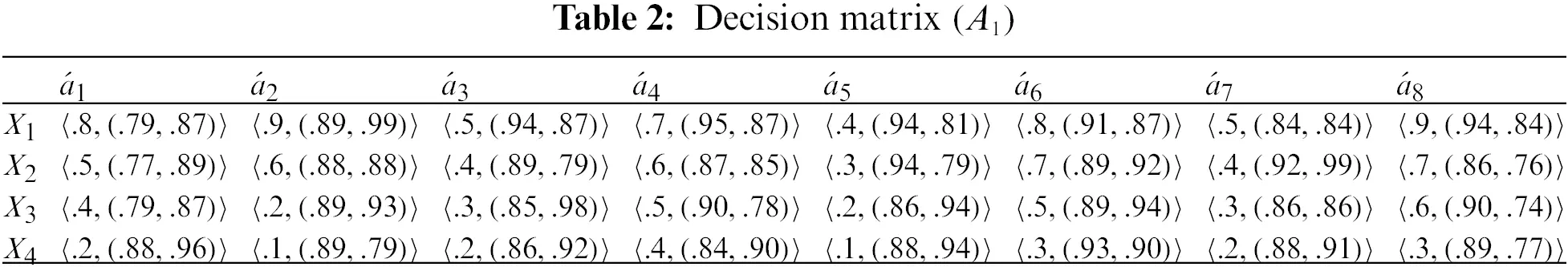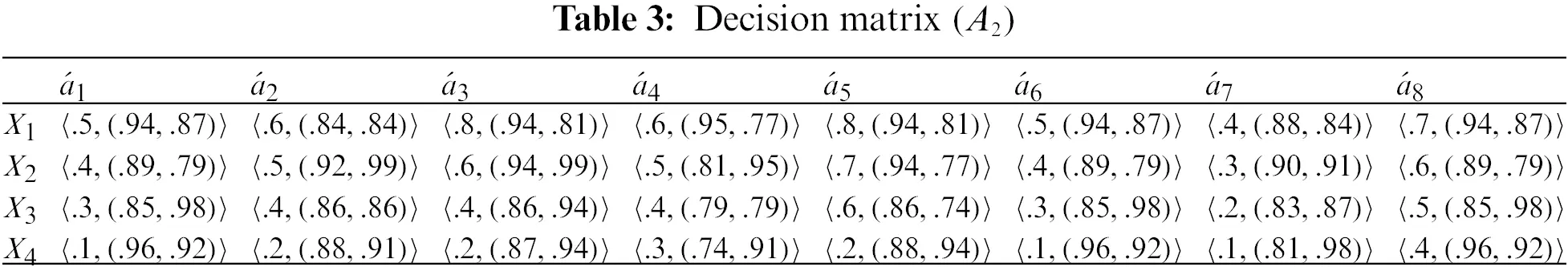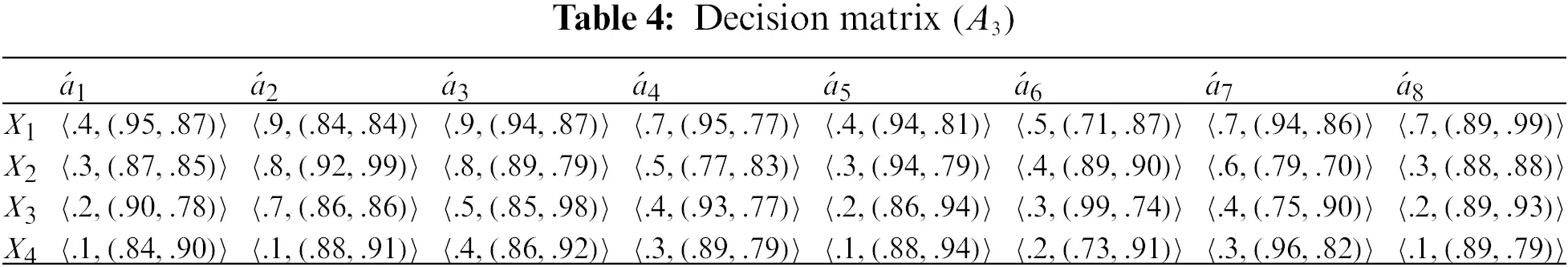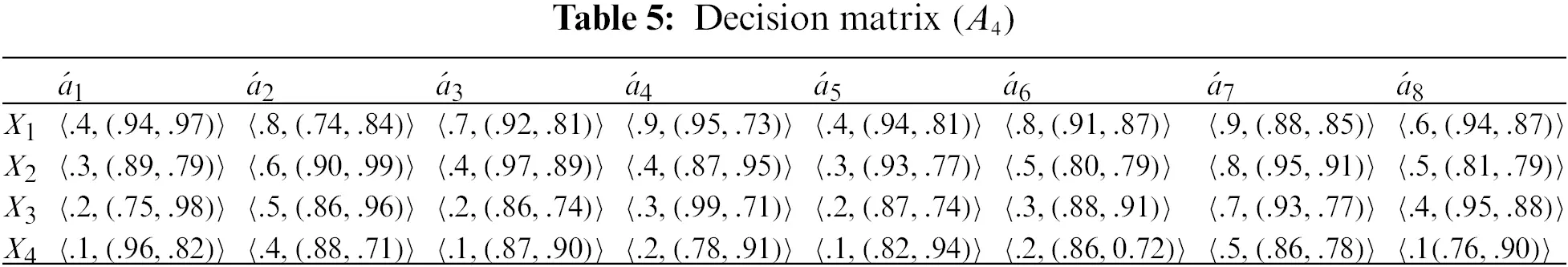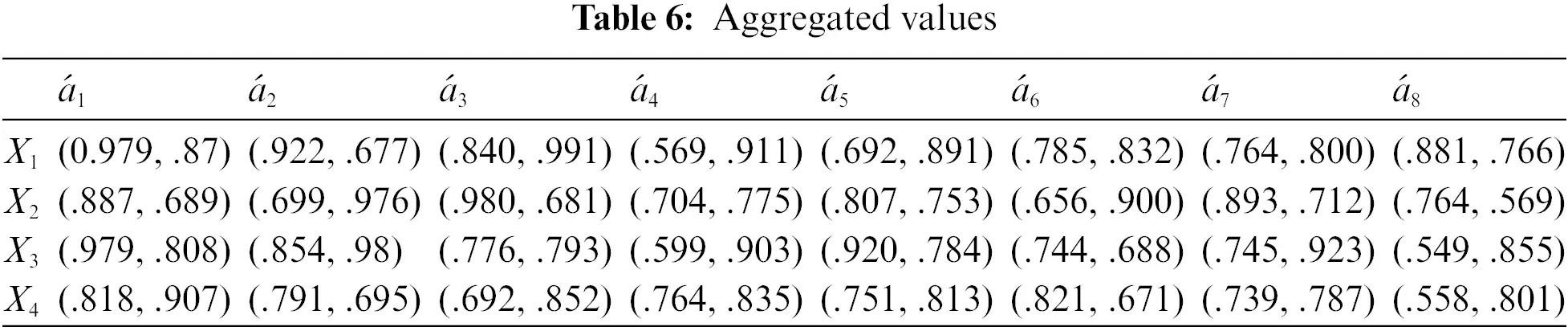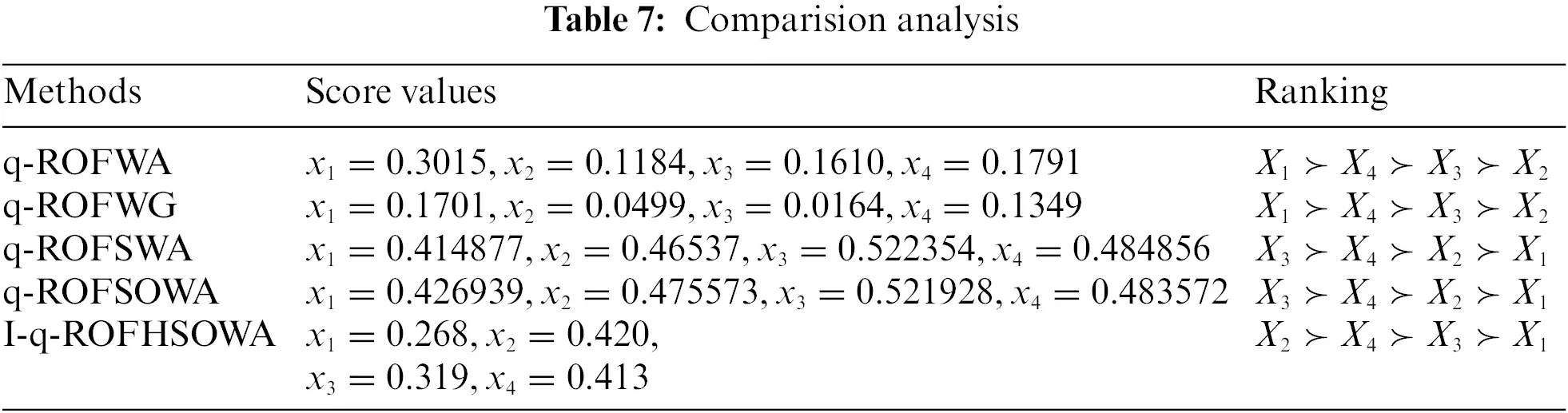Calculate the score value by using Definition 2.3, which is X1=0.268,X2=0.420,X3=0.319,X4=0.413.

5  Comparision Analysis

In this section, some current techniques under the existing structures, namely q-ROFS, q-ROFSS, and our proposed structure of q-ROFHSS have been compared in order to show the effectiveness of the proposed model. We have developed the MADM technique under the q-ROFHSOWA and I-q-ROFHSOWA operators. Our proposed technique can explain the most suitable results of DM problems. Since the proposed model is competent and adaptable, it can continue working hard despite any disruptions that may occur. The results obtained from the proposed techniques are different as compared to hybrid techniques. The results show that the operator of the proposed structure is more capable, reliable and effective. Fig. 1 shows a graphical ranking order of the alternatives to our proposed technique compared to the existing techniques.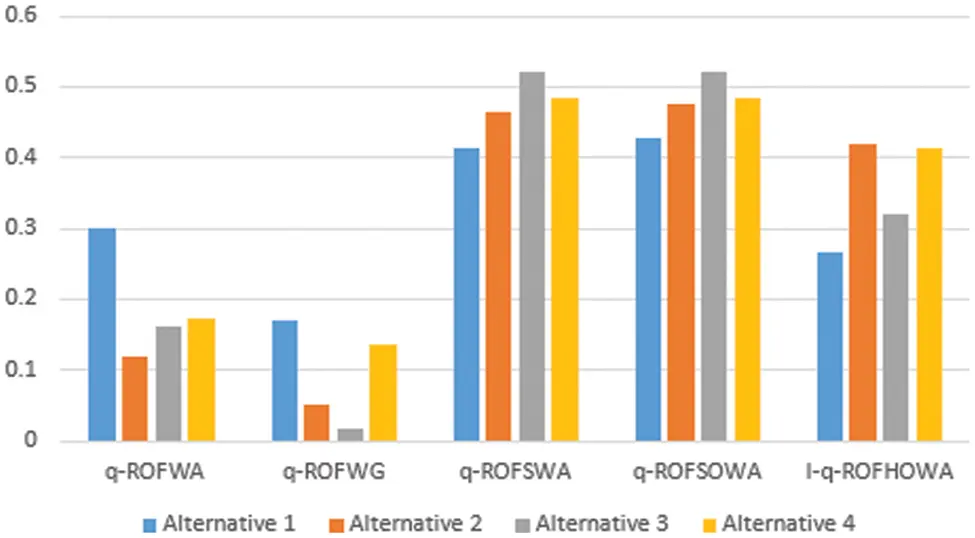Figure 1: Graphical representation of existing studies with proposed study

6  Conclusion

In this study, we developed a new decision-making technique under the q-rung orthopair fuzzy hypersoft environment with the induced ordered weighted averaging operator. We have initiated two types of operators i.e., ordered weighted averaging operator and induced ordered weighted averaging operator. The induced ordered weighted averaging operator is very helpful in decision-making problems with q-rung orthopair fuzzy hypersoft numbers under uncertain conditions. We have also studied some of its basic results and properties. All attribute values are considered by the method described in this article for selecting a real estate project based on the computation of aggregation operators from considered data in the form of membership and non-membership. Finally, we developed an application in an MADM problem. In this problem, we discussed the selection of the best real-estate project for investment. The induced ordered weighted averaging operator is very useful for this problem because this operator represents the uncertain data in the best form under q-rung orthopair fuzzy hypersoft numbers. This decision-making technique proves the information in the best way because in this process the decision-maker uses the order-inducing strategy. The main feature of the suggested strategy is that it offers a useful and adaptable way to effectively help the DM in uncertain situations. In addition, the proposed method can be used both for q-rung orthopair fuzzy hypersoft numbers and q-rung orthopair fuzzy soft numbers, which accurately represent the uncertainty. We plan to use the aggregation operator to solve DM problems in the future when dealing with more ambiguous data, including interval-valued q-rung orthopair fuzzy hypersoft sets, and interval-valued q-rung orthopair fuzzy hypersoft linguistic term sets.

Funding Statement: The authors received no specific funding for this study.

Conflicts of Interest: The authors declare that they have no conflicts of interest to report regarding the present study.

## References

1. Mishra, A. R., Rani, P., Krishankumar, R., Ravichandran, K., & Kar, S. (2021). An extended fuzzy decision-making framework using hesitant fuzzy sets for the drug selection to treat the mild symptoms of coronavirus disease 2019 (COVID-19). Applied Soft Computing, 103, 107155. [Google Scholar] [PubMed] [CrossRef]
2. Zadeh, L. A. (1965). Information and control. Fuzzy Sets, 8(3), 338-353. [Google Scholar]
3. Du, C., & Ye, J. (2021). Weighted parameterized correlation coefficients of indeterminacy fuzzy multisets and their multicriteria group decision making method with different decision risks. Computer Modeling in Engineering & Sciences, 129(1), 341-354. [Google Scholar] [CrossRef]
4. Atanassov, K. T. (1989). More on intuitionistic fuzzy sets. Fuzzy Sets and Systems, 33(1), 37-45. [Google Scholar] [CrossRef]
5. Atanassov, K. T. (1999). Intuitionistic fuzzy sets. In: Intuitionistic fuzzy sets. Studies in fuzziness and soft computing, physica, vol. 35, pp. 1–137. Heidelberg: Springer.
6. De, S. K., Biswas, R., & Roy, A. R. (2000). Some operations on intuitionistic fuzzy sets. Fuzzy Sets and Systems, 114(3), 477-484. [Google Scholar] [CrossRef]
7. De, S. K., Biswas, R., & Roy, A. R. (2001). An application of intuitionistic fuzzy sets in medical diagnosis. Fuzzy Sets and Systems, 117(2), 209-213. [Google Scholar] [CrossRef]
8. Szmidt, E., & Kacprzyk, J. (2000). Distances between intuitionistic fuzzy sets. Fuzzy Sets and Systems, 114(3), 505-518. [Google Scholar] [CrossRef]
9. Gulzar, M., Mateen, M. H., Alghazzawi, D., & Kausar, N. (2020). A novel applications of complex intuitionistic fuzzy sets in group theory. IEEE Access, 8, 196075-196085. [Google Scholar] [CrossRef]
10. Yager, R. R. (2013). Pythagorean membership grades in multicriteria decision making. IEEE Transactions on Fuzzy Systems, 22(4), 958-965. [Google Scholar] [CrossRef]
11. Peng, X., & Yang, Y. (2015). Some results for pythagorean fuzzy sets. International Journal of Intelligent Systems, 30(11), 1133-1160. [Google Scholar] [CrossRef]
12. Garg, H. (2018). Linguistic pythagorean fuzzy sets and its applications in multiattribute decision-making process. International Journal of Intelligent Systems, 33(6), 1234-1263. [Google Scholar] [CrossRef]
13. Yager, R. R. (2016). Generalized orthopair fuzzy sets. IEEE Transactions on Fuzzy Systems, 25(5), 1222-1230. [Google Scholar] [CrossRef]
14. Madasi, J. D., Khan, S., Kausar, N., Pamucar, D., & Gulistan, M. (2022). N-Cubic q-rung orthopair fuzzy sets: Analysis of the use of mobile app in the education sector. Computational Intelligence and Neuroscience, 2022, 1-24. [Google Scholar] [PubMed] [CrossRef]
15. Zulqarnain, R. M., Siddique, I., Iampan, A., & Baleanu, D. (2022). Aggregation operators for interval-valued pythagorean fuzzy soft set with their application to solve multi-attribute group decision making problem. Computer Modeling in Engineering & Sciences, 131(3), 1717-1750. [Google Scholar] [CrossRef]
16. Smarandache, F. (2018). Extension of soft set to hypersoft set, and then to plithogenic hypersoft set. Neutrosophic Sets and Systems, 22(1), 168-170. [Google Scholar]
17. Zulqarnain, R. M., Xin, X. L., & Saeed, M. (2021). A development of pythagorean fuzzy hypersoft set with basic operations and decision-making approach based on the correlation coefficient. Theory and Application of Hypersoft Set, 40(6), 149-168. [Google Scholar]
18. Siddique, I., Zulqarnain, R. M., Ali, R., Jarad, F., & Iampan, A. (2021). Multicriteria decision-making approach for aggregation operators of pythagorean fuzzy hypersoft sets. Computational Intelligence and Neuroscience, 2021, 2036506. [Google Scholar] [PubMed] [CrossRef]
19. Khan, S., Gulistan, M., & Wahab, H. A. (2022). Development of the structure of q-rung orthopair fuzzy hypersoft set with basic operations. Punjab University Journal of Mathematics, 53(12), 881-892. [Google Scholar]
20. Wei, G. (2010). Some induced geometric aggregation operators with intuitionistic fuzzy information and their application to group decision making. Applied Soft Computing, 10(2), 423-431. [Google Scholar] [CrossRef]
21. Xu, Y., & Wang, H. (2012). The induced generalized aggregation operators for intuitionistic fuzzy sets and their application in group decision making. Applied Soft Computing, 12(3), 1168-1179. [Google Scholar] [CrossRef]
22. Zulqarnain, R. M., Siddique, I., Ali, R., Jarad, F., & Iampan, A. (2021). Multicriteria decision-making approach for pythagorean fuzzy hypersoft sets interaction aggregation operators. Mathematical Problems in Engineering, 2021, 9964492. [Google Scholar] [CrossRef]
23. Liu, P., & Wang, P. (2018). Some q-rung orthopair fuzzy aggregation operators and their applications to multiple-attribute decision making. International Journal of Intelligent Systems, 33(2), 259-280. [Google Scholar] [CrossRef]
24. Peng, X., Dai, J., & Garg, H. (2018). Exponential operation and aggregation operator for q-rung orthopair fuzzy set and their decision-making method with a new score function. International Journal of Intelligent Systems, 33(11), 2255-2282. [Google Scholar] [CrossRef]
25. Liu, P., Mahmood, T., & Ali, Z. (2019). Complex q-rung orthopair fuzzy aggregation operators and their applications in multi-attribute group decision making. Information, 11(1), 5. [Google Scholar] [CrossRef]
26. Garg, H., Gwak, J., Mahmood, T., & Ali, Z. (2020). Power aggregation operators and vikor methods for complex q-rung orthopair fuzzy sets and their applications. Mathematics, 8(4), 538. [Google Scholar] [CrossRef]
27. Khan, S., Gulistan, M., Kausar, N., Kousar, S., & Pamucar, D. (2022). Analysis of cryptocurrency market by using q-rung orthopair fuzzy hypersoft set algorithm based on aggregation operators. Complexity, 2022, 7257449. [Google Scholar] [CrossRef]

Khan, S., Gulistan, M., Kausar, N., Pamucar, D., Hong, T. et al. (2023). Aggregation Operators for Decision Making Based on q-Rung Orthopair Fuzzy Hypersoft Sets: An Application in Real Estate Project. CMES-Computer Modeling in Engineering & Sciences, 136(3), 3141–3156.This work is licensed under a Creative Commons Attribution 4.0 International License , which permits unrestricted use, distribution, and reproduction in any medium, provided the original work is properly cited.

View

Like

## Related articles

• C. Jiang, X. Han, D. Li